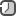### How to calculate confidence interval in R

A confidence interval for the population mean gives an indication of how accurately the sample mean estimates the population mean. A 95% confidence interval is defined as an interval calculated in such a way that if a large number of samples were drawn from a population and the interval calculated for each of these samples, 95% of the intervals will contain the true population mean value.A prediction interval gives an indication of how accurately the sample mean predicts the value of a further observation drawn from the population.

Confidence interval defines like: estimate ± margin of error.

A 95% confidence interval (CI) is twice the standard error (also called margin of error) plus or minus the mean. In our example, suppose the mean is 990 and standard deviation as computed is 47.4, then we would have a confidence interval (895.2, 1084.8) i.e. 990 ± 2 * 47. 4 . If we repeatedly choose many samples, each would have a different confidence interval but statistics tells us 95% of the time, CI will contain the true population mean. There are other stringent CIs like 99.7% but 95% is a golden standard for all practical purposes.

The simplest way to obtain a confidence interval for a sample mean is with the t.test function, which provides one with the output.

```x = rnorm(30, 195, 17)

t.test(rnorm(30, 195, 17))

One Sample t-test

data:  rnorm(30, 195, 17)
t = 59.174, df = 29, p-value < 2.2e-16
alternative hypothesis: true mean is not equal to 0

95 percent confidence interval:
190.1872 203.8047

sample estimates:
mean of x
196.9959
```

From the results, you can see that the mean is 196.99, with a 95% confidence interval of 190.18 to 203.804.